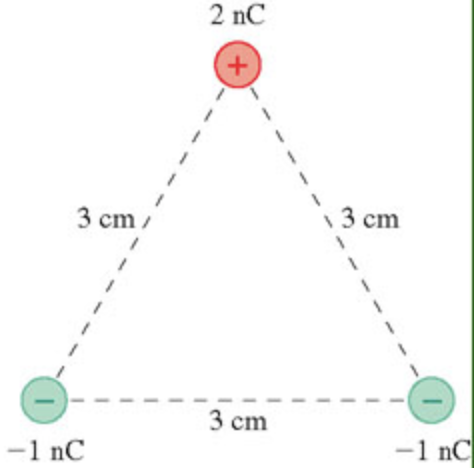In: Physics

# What is the electric potential energy of the group of charges in the figure?

What is the electric potential energy of the group of charges in the figure?## Solutions

##### Expert Solution

Concepts and reason

The concept used to solve this problem is electrical potential energy. Initially, draw the free body diagram for the group of charges forms an equilateral triangle to calculate the electric potential energy for the group of charges $$q_{12}, q_{23}$$ and $$q_{13}$$ by using the distance among those charges are $$r_{12}, r_{23}$$, and $$r_{13}$$. Finally, calculate the total electric potential for the group of charges mentioned in the figure.

Fundamentals

The expression for the coulomb's constant in terms of permittivity of free space is $$k=\frac{1}{4 \pi \varepsilon_{0}}$$

Here, $$\varepsilon_{0}$$ is the permittivity of free space. Electric potential energy for the group of point charges is defined as the "work required assembling this charges by bringing them close together from an infinite distance". The expression for the electric potential energy for the group of charges is, $$U=\left[\frac{k q_{1} q_{2}}{r_{12}}+\frac{k q_{2} q_{3}}{r_{23}}+\frac{k q_{1} q_{3}}{r_{13}}\right]$$

Here, $$q_{1}, q_{2}$$ and $$q_{3}$$ is the charges, $$k$$ is the coulomb's constant, and $$r_{12}, r_{23}$$ and $$r_{13}$$ is the distance between the those charges.

The expression for the coulomb's constant in terms of permittivity of free space is given by,

$$k=\frac{1}{4 \pi \varepsilon_{0}}$$

Here, $$\varepsilon_{0}$$ is the permittivity of free space. Substitute $$8.85 \times 10^{-12} \mathrm{C}^{2} \cdot \mathrm{N}^{-1} \cdot \mathrm{m}^{-2}$$ for $$\varepsilon_{0}$$ to find the coulomb's constant.

\begin{aligned} k=& \frac{1}{4(3.14)\left(8.85 \times 10^{-12} \mathrm{C}^{2} \cdot \mathrm{N}^{-1} \cdot \mathrm{m}^{-2}\right)} \\ &=9.0 \times 10^{9} \mathrm{~N} \cdot \mathrm{m}^{2} \cdot \mathrm{C}^{-2} \end{aligned}

The coulomb's constant is measured in terms of vacuum permittivity, the coulomb constant is also called electric force constant or electrostatic constant.

The expression for the electric potential energy is,

$$U=\left[\frac{k q 1 q 2}{r_{12}}+\frac{k q 2 q 3}{r_{23}}+\frac{k q 1 q 3}{r_{13}}\right]$$

Rearrange the expression as,

$$U=k\left[\frac{q_{1} q_{2}}{r 12}+\frac{q_{2} q_{3}}{r 23}+\frac{q_{1} q_{3}}{r 13}\right]$$

Substitute $$2 \mathrm{n} \mathrm{C}$$ for $$q_{1},-1 \mathrm{n} \mathrm{C}$$ for $$q_{2},-1 \mathrm{n} \mathrm{C}$$ for $$q_{3}, 3 \mathrm{~cm}$$ for $$r_{12}, r_{23}$$ and $$r_{13},$$ and $$9.0 \times 10^{9} \mathrm{~N} \cdot \mathrm{m}^{2} / \mathrm{C}^{2}$$ for $$k$$ to

find the electric potential energy.

$$\begin{array}{c} U=\left(9.0 \times 10^{9} \mathrm{~N} \cdot \mathrm{m}^{2} / \mathrm{C}^{2}\right)\left[\frac{(2 \mathrm{nC})(-1 \mathrm{nC})}{(3 \mathrm{~cm})}+\frac{(-1 \mathrm{n} \mathrm{C})(-1 \mathrm{n} \mathrm{C})}{(3 \mathrm{~cm})}+\frac{(2 \mathrm{nC})(-1 \mathrm{nC})}{(3 \mathrm{~cm})}\right] \frac{\left(\frac{10^{-9} \mathrm{C}}{1 \mathrm{nC}}\right)}{\left(\frac{10^{-2} \mathrm{~m}}{1 \mathrm{~cm}}\right)} \\ =\left(9.0 \times 10^{9} \mathrm{~N} \cdot \mathrm{m}^{2} / \mathrm{C}^{2}\right)\left[\frac{-2 \times 10^{-18} \mathrm{C}^{2}+1 \times 10^{-18} \mathrm{C}^{2}-2 \times 10^{-18} \mathrm{C}^{2}}{3 \times 10^{-2} \mathrm{~m}}\right] \\ =-9.0 \times 10^{-7} \mathrm{~J} \end{array}$$

The electric potential energy of the system of charges is $$-9.0 \times 10^{-7} \mathrm{~J}$$.

The net electric potential energy for the system of charges is calculated using the concept of equipotential surface; from the surface, the group of charges assembles to bring together from an infinite distance against the electric force.

The electric potential energy of the system of charges is $$-9.0 \times 10^{-7} \mathrm{~J}$$.